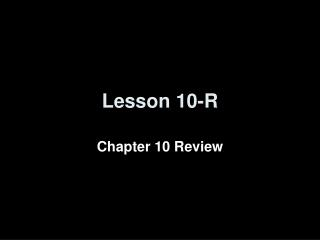Download PresentationLesson 10-R

# Lesson 10-R - PowerPoint PPT PresentationDownload Presentation## Lesson 10-R

- - - - - - - - - - - - - - - - - - - - - - - - - - - E N D - - - - - - - - - - - - - - - - - - - - - - - - - - -
##### Presentation Transcript

1. Lesson 10-R Chapter 10 Review

2. Objectives • Review Chapter 10 material

3. Parts of Circles • Circumference (Perimeter) • once around the outside of the circle; Formulas: C = 2πr = dπ • Chord • segment with endpoints of the edge of the circle • Radius • segment with one endpoint at the center and one at the edge • Diameter • segment with endpoints on the edge and passes thru the center • longest chord in a circle • is twice the length of a radius • Other parts • Center: is also the name of the circle • Secant: chord that extends beyond the edges of the circle • Tangent: a line (segment) that touches the circle at only one point

4. Arcs in Circles • Arc is the edge of the circle between two points • An arc’s measure = measure of its central angle • All arcs (and central angles) have to sum to 360° • If two arcs have the same measure then the chords that form those arcs have the same measure • If a radius is perpendicular to a chord then it bisects the chord and the arc formed by the chord (example arc AED below) • Major Arc (example: arc DAB) • measures more than 180° • more than ½ way around the circle • Minor Arc (example: arc AED) • measures less than 180° • less than ½ way around the circle • Semi-circle (example: arc EAB) • measures 180° • defined by a diameter BE is a diameter and AB = AD 120° B A C 120° 60° E D 60°

5. Angles Associated with Circles V K L minor arc LK = 10° minor arc NM = 70° minor arc FG = 110° minor arc EH = 36° minor arc BD = 110° B F E 10° A V M 36° N C C 110° 110° H C D G 70°

6. Segments Inside/Outside of Circles • Segments that intersect inside or outside the circle have the length of their parts defined by: Two ChordsInside a Circle Two SecantsFrom Outside Point Secant & Tangent From Outside Point J J K 4 3 5 6 L K K L 3 4 J T 9 8 7 11 6 N N M M M LJ · JM = NJ · JK 3  8 = 6  4 JT · JT = JK · JM 6  6 = 3  12 JL · JN = JK · JM 5  12 = 4  15 Inside the circle, it’s the parts of the chordsmultiplied together Outside the circle, it’s the outside part multiplied by the whole length OW = OW

7. Tangents and Circles • Tangents and radii always form a right angle • We can use the converse of the Pythagorean theorem to check if a segment is tangent • The distance from a point outside the circle along its two tangents to the circle is always the same distance Example 1 Given: JT is tangent to circle C JC = 25 and JT = 20 Find the radius Example 2 Given: same radius as example 1 JC = 25 and JS = 16 Is JS tangent to circle C? J S T JC² = JT² + TC² 25² = 20² + r² 625 = 400 + r² 225 = r² 15 = r JC² = JS² + SC² 25² = 16² + 15² 625 = 256 + 225 625 ≠ 481 JS is not tangent C

8. Equation of Circles • A circle’s algebraic equation is defined by: (x – h)² + (y – k)² = r² where the point (h, k) is the location of the center of the circleand r is the radius of the circle • Circles are all points that are equidistant (that is the distance of the radius) from a central point (the center)

9. Summary & Homework • Summary: • A • Homework: • study for the test